# cyclebasis

Fundamental cycle basis of graph

## Syntax

``cycles = cyclebasis(G)``
``[cycles,edgecycles] = cyclebasis(G)``

## Description

example

````cycles = cyclebasis(G)` computes the fundamental cycle basis of an undirected graph. The output `cycles` is a cell array that indicates which nodes belong to each fundamental cycle. ```

example

````[cycles,edgecycles] = cyclebasis(G)` also returns the edges in each cycle. The output `edgecycles` is a cell array where `edgecycles{k}` gives the edges between the nodes in `cycles{k}`.```

## Examples

collapse all

Create and plot an undirected graph.

```s = [1 1 2 2 3 4 4 5 5 6 7 8]; t = [2 4 3 5 6 5 7 6 8 9 8 9]; G = graph(s,t); plot(G)```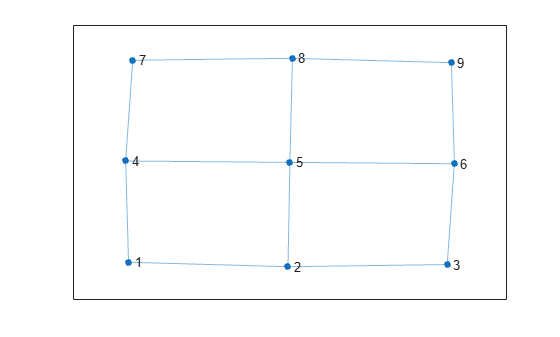Calculate which nodes are in each fundamental cycle.

`cycles = cyclebasis(G)`
```cycles=4×1 cell array {[1 2 5 4]} {[2 3 6 5]} {[4 5 8 7]} {[5 6 9 8]} ```

Compute the nodes and edges in the fundamental cycles of a graph, visualize the fundamental cycles, and then use the fundamental cycles to find other cycles in the graph.

Create and plot an undirected graph.

```s = [1 1 1 2 2 3 3 4 4 4 5 6]; t = [2 3 4 4 5 4 6 5 6 7 7 7]; G = graph(s,t); plot(G);```Compute the fundamental cycle basis of the graph.

`[cycles,edgecycles] = cyclebasis(G)`
```cycles=6×1 cell array {[1 2 4]} {[1 3 4]} {[2 4 5]} {[3 4 6]} {[4 5 7]} {[4 6 7]} ```
```edgecycles=6×1 cell array {[ 1 4 3]} {[ 2 6 3]} {[ 4 8 5]} {[ 6 9 7]} {[8 11 10]} {[9 12 10]} ```

Highlight each of the fundamental cycles, using `tiledlayout` and `nexttile` to construct an array of subplots. For each subplot, first get the nodes of the corresponding cycle from `cycles`, and the edges from `edgecycles`. Then, plot the graph and highlight those nodes and edges.

```tiledlayout flow for k = 1:length(cycles) nexttile highlight(plot(G),cycles{k},'Edges',edgecycles{k},'EdgeColor','r','NodeColor','r') title("Cycle " + k) end```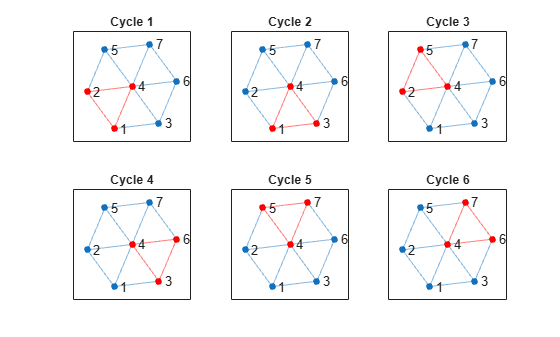You can construct any other cycle in the graph by finding the symmetric difference between two or more fundamental cycles using the `setxor` function. For example, take the symmetric difference between the first two cycles and plot the resulting new cycle.

```figure new_cycle_edges = setxor(edgecycles{1},edgecycles{2}); highlight(plot(G),'Edges',new_cycle_edges,'EdgeColor','r','NodeColor','r')```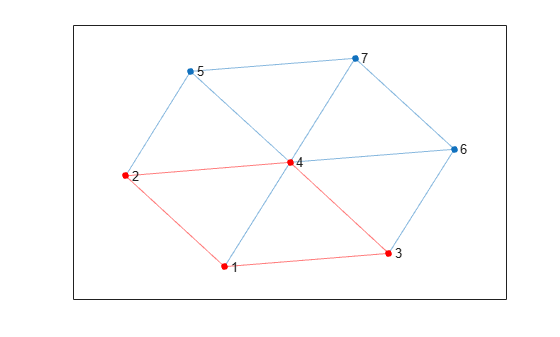While every cycle can be constructed by combining cycles from the cycle basis, not every combination of basis cycles forms a valid cycle.

Examine how the outputs of the `cyclebasis` and `allcycles` functions scale with the number of edges in a graph.

Create and plot a square grid graph with three nodes on each side of the square.

```n = 5; A = delsq(numgrid('S',n)); G = graph(A,'omitselfloops'); plot(G)```Compute all cycles in the graph using `allcycles`. Use the `tiledlayout` function to construct an array of subplots and highlight each cycle in a subplot. The results indicate there are a total of 13 cycles in the graph.

```[cycles,edgecycles] = allcycles(G); tiledlayout flow for k = 1:length(cycles) nexttile highlight(plot(G),cycles{k},'Edges',edgecycles{k},'EdgeColor','r','NodeColor','r') title("Cycle " + k) end```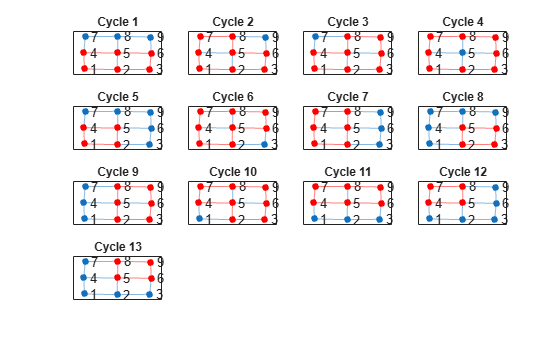Some of these cycles can be seen as combinations of smaller cycles. The `cyclebasis` function returns a subset of the cycles that form a basis for all other cycles in the graph. Use `cyclebasis` to compute the fundamental cycle basis and highlight each fundamental cycle in a subplot. Even though there are 13 cycles in the graph, there are only four fundamental cycles.

```[cycles,edgecycles] = cyclebasis(G); tiledlayout flow for k = 1:length(cycles) nexttile highlight(plot(G),cycles{k},'Edges',edgecycles{k},'EdgeColor','r','NodeColor','r') title("Cycle " + k) end```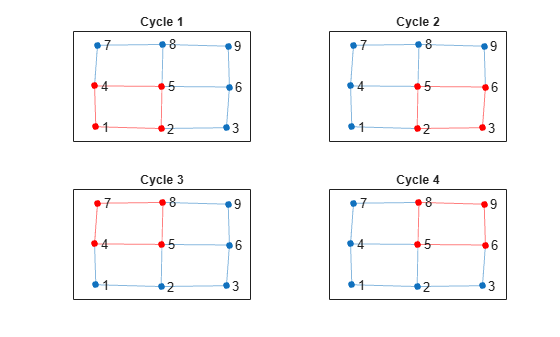Now, increase the number of nodes on each side of the square graph from three to four. This represents a small increase in the size of the graph.

```n = 6; A = delsq(numgrid('S',n)); G = graph(A,'omitselfloops'); figure plot(G)```Use `allcycles` to compute all of the cycles in the new graph. For this graph there are over 200 cycles, which is too many to plot.

`allcycles(G)`
```ans=213×1 cell array {[ 1 2 3 4 8 7 6 5]} {[ 1 2 3 4 8 7 6 10 9 5]} {[1 2 3 4 8 7 6 10 11 12 16 15 14 13 9 5]} {[ 1 2 3 4 8 7 6 10 11 15 14 13 9 5]} {[ 1 2 3 4 8 7 6 10 14 13 9 5]} {[ 1 2 3 4 8 7 11 10 6 5]} {[ 1 2 3 4 8 7 11 10 9 5]} {[ 1 2 3 4 8 7 11 10 14 13 9 5]} {[ 1 2 3 4 8 7 11 12 16 15 14 10 6 5]} {[ 1 2 3 4 8 7 11 12 16 15 14 10 9 5]} {[ 1 2 3 4 8 7 11 12 16 15 14 13 9 5]} {[1 2 3 4 8 7 11 12 16 15 14 13 9 10 6 5]} {[ 1 2 3 4 8 7 11 15 14 10 6 5]} {[ 1 2 3 4 8 7 11 15 14 10 9 5]} {[ 1 2 3 4 8 7 11 15 14 13 9 5]} {[ 1 2 3 4 8 7 11 15 14 13 9 10 6 5]} ⋮ ```

Despite the large number of cycles in the graph, `cyclebasis` still returns a small number of fundamental cycles. Each of the cycles in the graph can be constructed using only nine fundamental cycles.

```[cycles,edgecycles] = cyclebasis(G); figure tiledlayout flow for k = 1:length(cycles) nexttile highlight(plot(G),cycles{k},'Edges',edgecycles{k},'EdgeColor','r','NodeColor','r') title("Cycle " + k) end```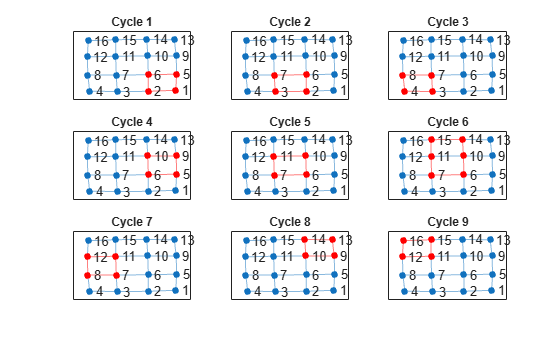The large increase in the number of cycles with only a small change in the size of the graph is typical for some graph structures. The number of cycles returned by `allcycles` can grow exponentially with the number of edges in the graph. However, the number of cycles returned by `cyclebasis` can, at most, grow linearly with the number of edges in the graph.

## Input Arguments

collapse all

Input graph, specified as a `graph` object. Use `graph` to create an undirected graph object.

Example: `G = graph(1,2)`

## Output Arguments

collapse all

Fundamental graph cycles, returned as a cell array. Each cell `cycles{k}` contains the nodes that belong to one of the fundamental cycles of `G`. Each cycle begins with the smallest node index. If `G` does not contain any cycles, then `cycles` is empty.

Every cycle in `G` is a combination of the fundamental cycles returned in `cycles`. If an edge is part of a cycle in `G`, then it is also part of at least one fundamental cycle in `cycles`.

The data type of the cells in `cycles` depends on whether the input graph contains node names:

• If graph `G` does not have node names, then each cell `cycles{k}` is a numeric vector of node indices.

• If graph `G` has node names, then each cell `cycles{k}` is a cell array of character vector node names.

Edges in each fundamental cycle, returned as a cell array. Each cell `edgecycles{k}` contains the edge indices for edges in cycle `cycles{k}`. If `G` does not contain any cycles, then `edgecycles` is empty.

collapse all

### Fundamental Cycle Basis

In undirected graphs, the fundamental cycle basis is a set of simple cycles that forms a basis for the cycle space of the graph. That is, any cycle in the graph can be constructed from the fundamental cycles. For an example, see Nodes and Edges in Fundamental Cycles.

The fundamental cycle basis of a graph is calculated from a minimum spanning tree of the graph. For each edge that is not in the minimum spanning tree, there exists one fundamental cycle which is composed of that edge, its end nodes, and the path in the minimum spanning tree that connects them.

The minimum spanning tree used in `cyclebasis` is generally different from the one returned by `minspantree`. It is chosen such that the cycles are short. However, `cyclebasis` is not guaranteed to return the shortest possible fundamental cycle basis.